Computational & Technology Resources an online resource for computational,engineering & technology publications not logged in - login Civil-Comp ProceedingsISSN 1759-3433 CCP: 84PROCEEDINGS OF THE FIFTH INTERNATIONAL CONFERENCE ON ENGINEERING COMPUTATIONAL TECHNOLOGY Edited by: B.H.V. Topping, G. Montero and R. Montenegro Paper 4An Adaptive Mesh Method for Hyperbolic Conservation Laws J. Felcman1 and P. Kubera21Faculty of Mathematics and Physics, Charles University, Prague, Czech Republic 2Faculty of Science, Purkyne University, Ústí nad Labem, Czech Republic doi:10.4203/ccp.84.4 Full Bibliographic Reference for this paper J. Felcman, P. Kubera, "An Adaptive Mesh Method for Hyperbolic Conservation Laws", in B.H.V. Topping, G. Montero, R. Montenegro, (Editors), "Proceedings of the Fifth International Conference on Engineering Computational Technology", Civil-Comp Press, Stirlingshire, UK, Paper 4, 2006. doi:10.4203/ccp.84.4 Keywords: mesh adaptation, geometric conservation law, finite volume method, moving discontinuities, Euler equations. Summary The subject-matter of this paper is the numerical simulation of the multidimensional inviscid compressible transonic gas flow in channels, past airfoils and cascades of profiles. The adaptive strategy is applied to the numerical solution of problem governed by hyperbolic partial differential equations. An adaptive mesh is constructed in the framework of the cell-centred finite volume scheme. An anisotropic mesh adaptation strategy is followed by a recovery of the approximate solution on the new mesh. The main feature of the proposed method is to keep the mass conservation of the numerical solution at each adaptation step. This allows the solution of the non-stationary problems. The geometric conservation law is employed. The numerical solution for the case of a non-stationary discontinuity wave is presented. We consider the flow of an inviscid perfect gas in a bounded domain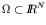and time interval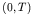with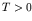. Here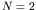or 3 for two- or three-dimensional flow, respectively, and we suppose that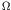is polygonal in two dimensions or polyhedral in three dimensions, respectively. Further we suppose, that the flow is adiabatic and we neglect the outer volume force. Our goal is to solve numerically the initial-boundary value problem described by the Euler equations. As a discrete analogy of the continuous problem we use the ADER higher-order finite volume scheme based on a polynomial reconstruction of a piecewise constant finite volume solution. For the study of the numerical order of accuracy of the proposed finite volume scheme see [1,2] and references therein. In  a time marching finite volume method for non-stationary problems was proposed and tested in for one-dimensional case. Here we present its further development and two-dimensional results. The algorithm is generally formulated in three dimensions. It consists of three basic sections at each time step: the time evolution of the numerical solution, the mesh adaptation and the recomputing of the numerical solution from the mesh before the adaptation to the mesh after the adaptation. In one time step the finite volume scheme is evaluated twice. Firstly for the prediction how to adapt the mesh, further for the update of the numerical solution itself. In the prediction part, we forecast the evolution of the numerical solution and adapt the mesh. The anisotropic mesh adaptation (AMA) is applied. For its description see e.g. . In  the necessary condition for the properties of the N-simplicial mesh, on which the discretization error is below the prescribed tolerance, is formulated. It is shown, how to control this necessary condition by the interpolation error and the anisotropic mesh adaptation technique is applied. For two- and three-dimensional numerical examples see e.g. . In the AMA technique, the equilateral mesh is constructed in the least squares sense. The length of an edge of an N-simplicial mesh is measured in the numerical solution dependent Riemann norm. After the adaptive mesh is constructed it is necessary to recompute the solution on the old mesh to its recovery on the newly adapted mesh. According to  the geometric mass conservation law has to be satisfied in this computational step. The perturbation method from  is applied. The two-dimensional numerical example employing the described higher-order method with proposed mesh adaptation is presented. The numerical aspects of the method are demonstrated with the example of the two-dimensional channel flow described by the Euler equations equipped with the discontinuous initial condition. The mesh adaptation corresponding to the various time instants is depicted. The proposed non-stationary mesh adaptation can help to improve the numerical results of a cell centered finite volume method. Numerical tests in progress show that it prevents the numerical solution from spurious oscillations. The proposed adaptation is value for the sufficiently precise solution despite increased computational time. References 1 J. Felcman, K. Findejs, "Higher-order finite volume method for multidimensional compressible flow", in "Proceedings of the International Conference on Numerical Analysis and Applied Mathematics", T. E. Simos, (Editor), WILEY-VCH, Weinheim, 186-189, 2005. 2 E. F. Toro, R. C. Millington, L. A. M. Nejad, "Towards very high order Godunov schemes", in "Godunov methods; Theory and applications", E. F. Toro, (Editor), Kluwer Academic Plenum Publishers, Basel, 907-940, 2001. 3 J. Felcman, P. Kubera, "Mesh adaptation for a time marching procedure", in "Numerical Mathematics and Advanced Applications ENUMATH 2005", A. Bermúdez, (Editor), Springer, Berlin, 2005. (accepted) 4 V. Dolejší, J. Felcman, "Anisotropic mesh adaptation for numerical solution of boundary value problems", Numerical Methods for Partial Differential Equations, 576-608, 2003. doi:10.1002/num.10104 5 J. Felcman, V. Dolejší, M. Feistauer, "Towards Adaptive Methods for the Modelling of the Compressible Flows", Journal of the Applied Mathematics, Statistics and Informatics, (1), 2, 5-32, 2005. 6 H. Tang, T. Tang, "Adaptive mesh methods for one- and two-dimensional hyperbolic conservation laws", SIAM J. Appl. Math., 487-515, 2003. doi:10.1137/S003614290138437X purchase the full-text of this paper (price £20) Back to top ©Civil-Comp Limited 2020 - terms & conditions